# Catch up on the latest AI articles# Using Contrastive Learning For Image Conversion Between Different Domains ~DCLGAN

3 main points
✔️ Developed a new GAN (DCLGAN) that combines contrast learning and Dual Learning
✔️ Address mode collapse by making minor changes to the loss function of DCLGAN
✔️ Achieve good quality image transformations even across widely different domains

(Submitted on 15 Apr 2021)
Accepted to NTIRE, CVPRW 2021
Subjects: Computer Vision and Pattern Recognition (cs.CV); Image and Video Processing (eess.IV)code：The images used in this article are from the paper, the introductory slides, or were created based on them.

## background

Image-to-Image Translation is a task that aims to convert an image from one domain to another. There are many examples, such as converting an image of a horse to an image of a zebra, a low-resolution image to a high-resolution image, or a photograph to a painting.

Among them, the problem setting where no correspondence between images is given is called unsupervised Image-to-Image Translation. In the unsupervised setting, the training is prone to instability due to the possibility of multiple possible mappings between domains. CycleGAN stabilizes training by restricting the possible mappings by considering the inverse transformation from the destination domain to the source domain and is known as a model that assumes Cycle consistency.

However, the Cycle Consistency-based model has a problem in that it cannot generate images in which the geometric structure in the image changes significantly. CUT achieves unsupervised image-to-image translation by maximizing the amount of mutual information between input and output patches. CUT achieves better performance than the conventional cycle-consistency-based models.

In the paper presented in this paper, we propose a new training method that combines contrastive learning with dual learning and a solution for mode collapse that was not addressed in CUT.

## DCLGAN

The authors named the newly proposed model DCLGAN, where DCL stands for Dual Contrastive Learning. Let's start with the overall picture of DCLGAN.

The following figure shows an overview of DCLGAN, which uses an architecture similar to CycleGAN, consisting of two Generators that perform bi-directional transformations between domains and two Discriminators that determine whether an image is real or not for each domain The following example shows how to use

DCLGAN further adds a multilayer perceptron like $H$ in the figure to project image patches to feature vectors.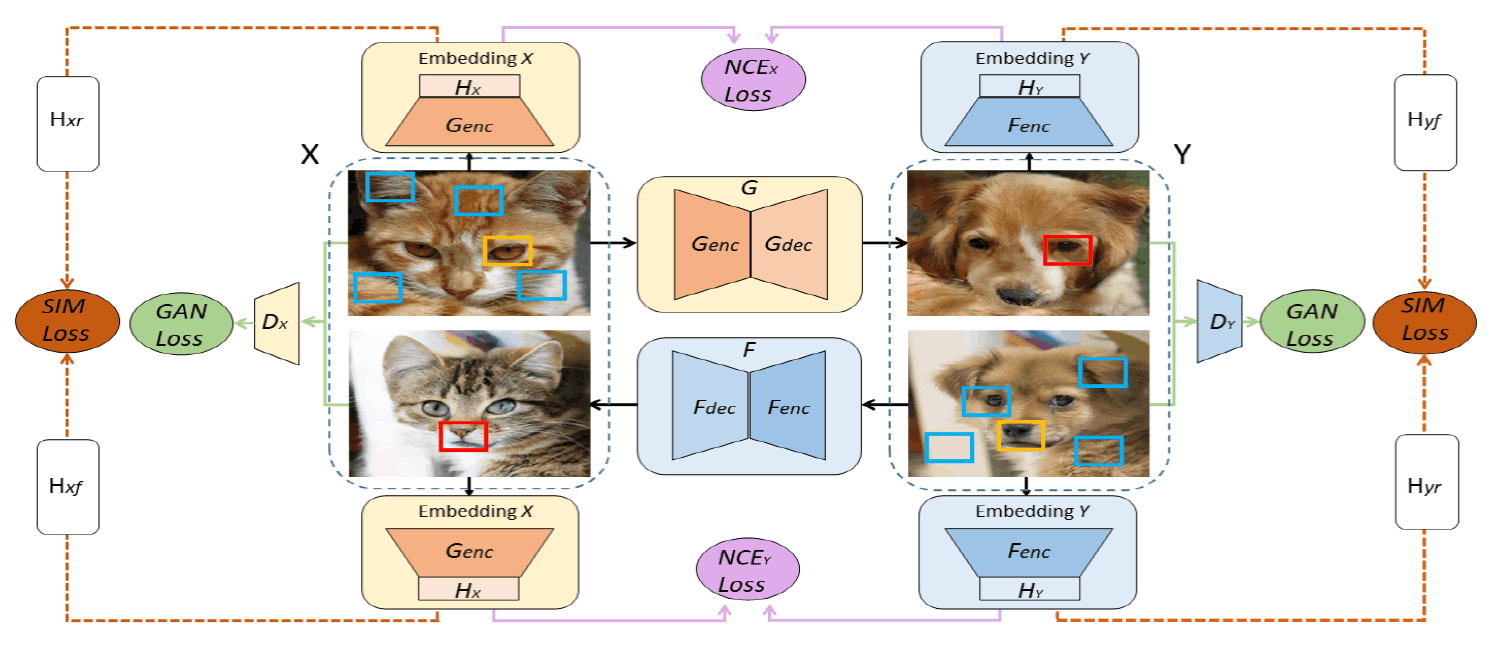The loss function expressed in the following equation is used to train DCLGAN.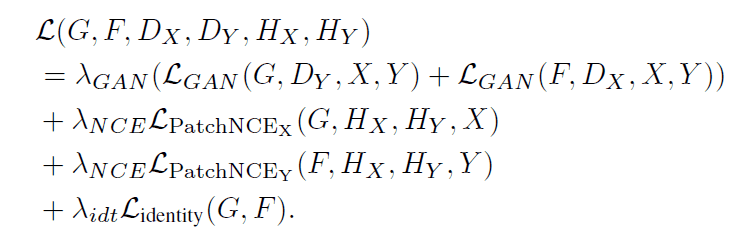This loss function consists of three types of losses $\mathcal{L}_{GAN},\mathcal{L}_{PatchNCE},\mathcal{L}_{identity}$. Let's look at each loss in detail.

The $\mathcal{L}_{GAN}$ is the loss used in traditional GAN and is the term needed for the Generator to generate images that the Discriminator cannot discriminate.

$\mathcal{L}_{PatchNCE}$ is the loss to maximize the mutual information content between the input and output images. It is also used in the CUT of the existing model. The specific calculation method is as follows.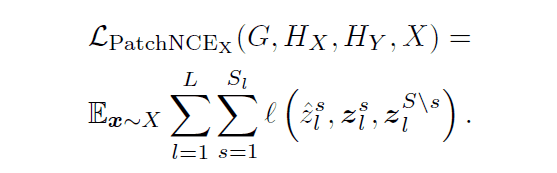In the equation $\hat{z}_l^s$ is the feature vector of the image patch obtained by transforming from domain $X$ to domain $Y$ (query), $z_l^s$ is the feature vector of the transformed source image patch corresponding to the query (positive example), and $\mathbb{z}_l^{S\backslash s}$ is the feature vector of the image patch in the transformed image patches that do not correspond to the query in the source image (negative example). In the above schematic, the query corresponds to the image patch surrounded by red squares, the positive example corresponds to the image patch surrounded by orange squares, and the negative example corresponds to the image patch surrounded by light blue squares.

The function $l$ in the equation is the function defined in the equation below. Cosine similarity is used for the function $sim$ in the equation.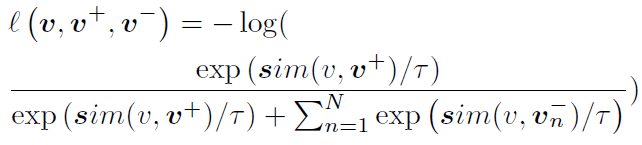Adding $\mathcal{L}_{PatchNCE}$ has the effect of pulling the query and positive example feature vectors together and pushing the query and negative example feature vectors apart.

Finally, $\mathcal{L}_{identity}$ is a loss defined by the following equation. This loss was also introduced in CycleGAN and aims to minimize the L1 distance between input and output images to avoid generating images with drastically different color compositions.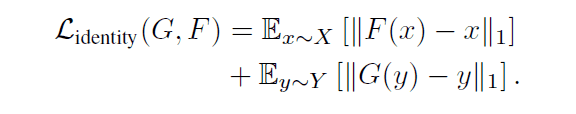These are the loss functions of DCLGAN, and our first impression is that DCLGAN combines the architecture of CycleGAN with the objective function of CUT. The unique feature of DCLGAN is that it uses dual learning to provide two encoders instead of a single encoder for the two domains in the latent space.

## SimDCL

The authors have further improved DCLGAN and proposed a model called SimDCL, which adds loss to the DCLGAN loss function to account for the similarity between images belonging to the same domain.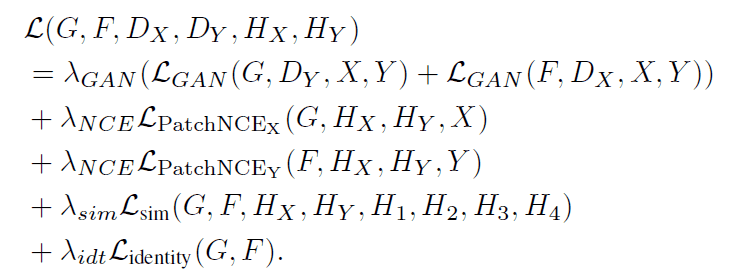The $\mathcal{L}_{sim}$ is the feature vector extracted by the encoder and the multilayer perceptron $H$, which is further transformed into a 64-dimensional feature vector by another lightweight network, and the L1 distance between vectors belonging to the same domain is calculated, which is expressed by where $x,y$ is the domain to which the image belongs and $r,f$ is a subscript indicating whether the image is real or generated.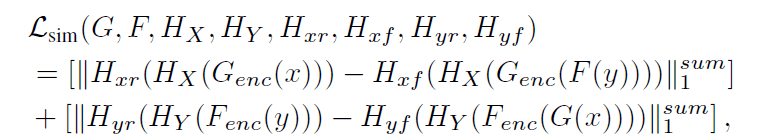This explicitly adds to the loss that images belonging to the same domain have some similar characteristics.

## Image Conversion Result

The authors first compared the two models, DCLGAN and SimDCL, with existing unsupervised Image-to-Image studies. Six datasets were used: Horse⇔Zebra, Cat⇔Dog, CityScapes, Van Gogh⇔Photo, Label⇔Facade, and Orange⇔Apple, and the evaluation metric was Frechet Inception Distance (FID).

The following table shows the results of all baseline and comparisons.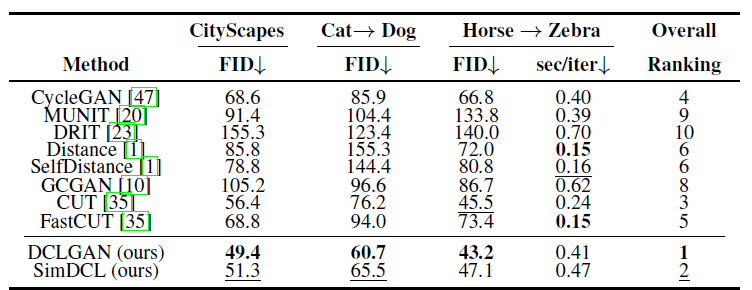DCLGAN achieves the best FID on the three datasets, and the training speed is also relatively fast, only slightly slower than CycleGAN.

The following table shows the results of further evaluation by selecting the top four methods.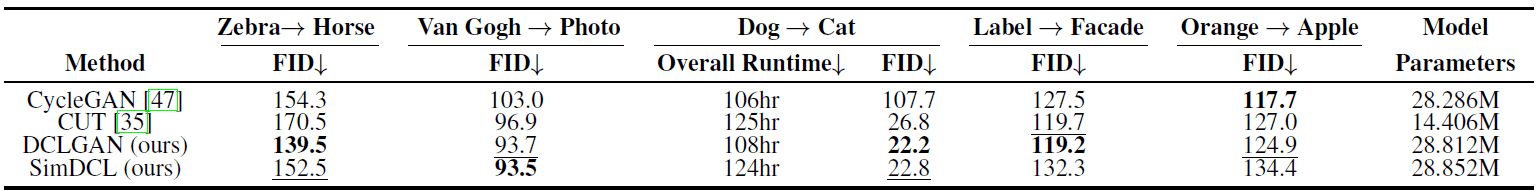We can see that DCLGAN performs best in most cases even with different datasets; SimDCL performs worse than DCLGAN in most cases but performs best for some tasks.

Let's look at the actual generated images for each dataset.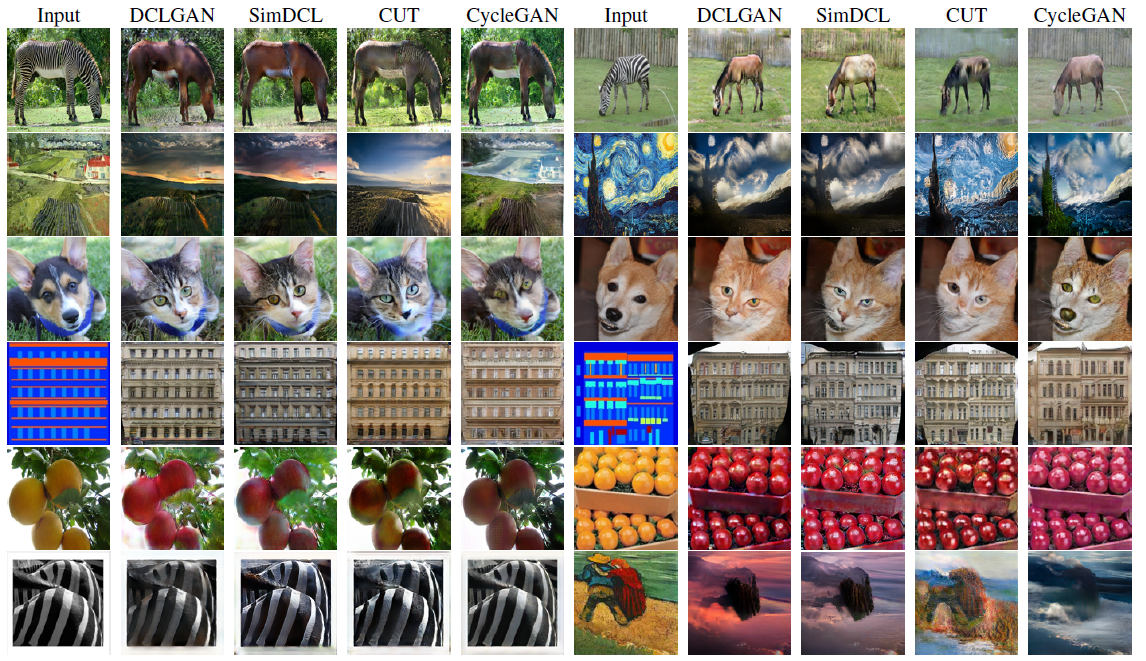The most notable feature of DCLGAN is the Dog⇒Cat conversion, whereas the other methods produce images that are either too broken or too canine-like. The other methods are too restrictive on the mapping, so if the structure of the image changes significantly before and after the conversion, the DCLGAN method cannot generate a good image. The Cycle Consistency-based method is too restrictive on the mapping, so it may not be able to produce a good image if the structure changes significantly before and after the transformation. On the other hand, CycleGAN produces good results when the color structure is changed from Orange to Apple. To emphasize the comparison with CUT, we also checked whether mode collapse occurs in the top four methods.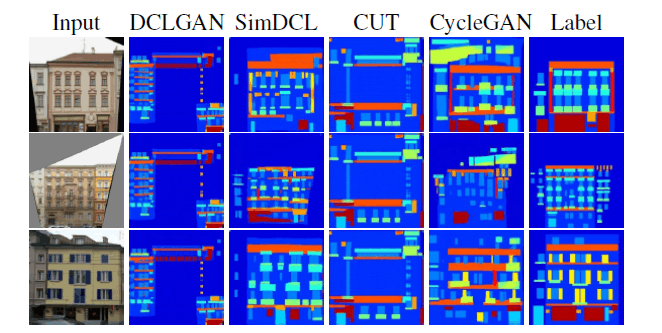All methods except SimDCL and CycleGAN output almost the same image regardless of the input. We also claim that SimDCL is the method that transforms the data closest to the correct data.

## Finally

I was very happy with the results of the simulation, but it was not clear why SimGAN with similarity loss suppressed the mode collapse. I was a little bit confused.

I wonder how much the difference in the number of parameters affects the quality of the generated image in comparison with CUT.

Will more models not use Cycle consistency in the future? It will be interesting to see how this develops in the future.

If you have any suggestions for improvement of the content of the article,